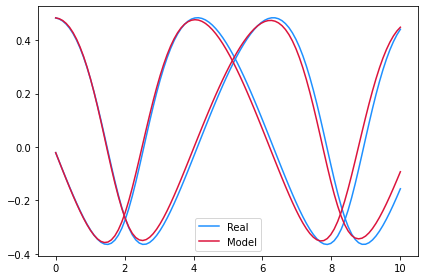# Neural ODE¤

This example trains a Neural ODE to reproduce a toy dataset of nonlinear oscillators.

This example is available as a Jupyter notebook here.

import time

import diffrax
import equinox as eqx  # https://github.com/patrick-kidger/equinox
import jax
import jax.nn as jnn
import jax.numpy as jnp
import jax.random as jrandom
import matplotlib.pyplot as plt
import optax  # https://github.com/deepmind/optax


We use Equinox to build neural networks. We use Optax for optimisers (Adam etc.)

Recalling that a neural ODE is defined as

$$y(t) = y(0) + \int_0^t f_\theta(s, y(s)) ds$$,

then here we're now about to define the $$f_\theta$$ that appears on that right hand side.

class Func(eqx.Module):
mlp: eqx.nn.MLP

def __init__(self, data_size, width_size, depth, *, key, **kwargs):
super().__init__(**kwargs)
self.mlp = eqx.nn.MLP(
in_size=data_size,
out_size=data_size,
width_size=width_size,
depth=depth,
activation=jnn.softplus,
key=key,
)

def __call__(self, t, y, args):
return self.mlp(y)


Here we wrap up the entire ODE solve into a model.

class NeuralODE(eqx.Module):
func: Func

def __init__(self, data_size, width_size, depth, *, key, **kwargs):
super().__init__(**kwargs)
self.func = Func(data_size, width_size, depth, key=key)

def __call__(self, ts, y0):
solution = diffrax.diffeqsolve(
diffrax.ODETerm(self.func),
diffrax.Tsit5(),
t0=ts,
t1=ts[-1],
dt0=ts - ts,
y0=y0,
stepsize_controller=diffrax.PIDController(rtol=1e-3, atol=1e-6),
saveat=diffrax.SaveAt(ts=ts),
)
return solution.ys


Toy dataset of nonlinear oscillators. Sample paths look like deformed sines and cosines.

def _get_data(ts, *, key):
y0 = jrandom.uniform(key, (2,), minval=-0.6, maxval=1)

def f(t, y, args):
x = y / (1 + y)
return jnp.stack([x, -x], axis=-1)

solver = diffrax.Tsit5()
dt0 = 0.1
saveat = diffrax.SaveAt(ts=ts)
sol = diffrax.diffeqsolve(
diffrax.ODETerm(f), solver, ts, ts[-1], dt0, y0, saveat=saveat
)
ys = sol.ys
return ys

def get_data(dataset_size, *, key):
ts = jnp.linspace(0, 10, 100)
key = jrandom.split(key, dataset_size)
ys = jax.vmap(lambda key: _get_data(ts, key=key))(key)
return ts, ys

def dataloader(arrays, batch_size, *, key):
dataset_size = arrays.shape
assert all(array.shape == dataset_size for array in arrays)
indices = jnp.arange(dataset_size)
while True:
perm = jrandom.permutation(key, indices)
(key,) = jrandom.split(key, 1)
start = 0
end = batch_size
while end < dataset_size:
batch_perm = perm[start:end]
yield tuple(array[batch_perm] for array in arrays)
start = end
end = start + batch_size


Main entry point. Try runnning main().

def main(
dataset_size=256,
batch_size=32,
lr_strategy=(3e-3, 3e-3),
steps_strategy=(500, 500),
length_strategy=(0.1, 1),
width_size=64,
depth=2,
seed=5678,
plot=True,
print_every=100,
):
key = jrandom.PRNGKey(seed)
data_key, model_key, loader_key = jrandom.split(key, 3)

ts, ys = get_data(dataset_size, key=data_key)
_, length_size, data_size = ys.shape

model = NeuralODE(data_size, width_size, depth, key=model_key)

# Training loop like normal.
#
# Only thing to notice is that up until step 500 we train on only the first 10% of
# each time series. This is a standard trick to avoid getting caught in a local
# minimum.

y_pred = jax.vmap(model, in_axes=(None, 0))(ti, yi[:, 0])
return jnp.mean((yi - y_pred) ** 2)

@eqx.filter_jit
def make_step(ti, yi, model, opt_state):
return loss, model, opt_state

for lr, steps, length in zip(lr_strategy, steps_strategy, length_strategy):
opt_state = optim.init(eqx.filter(model, eqx.is_inexact_array))
_ts = ts[: int(length_size * length)]
_ys = ys[:, : int(length_size * length)]
for step, (yi,) in zip(
):
start = time.time()
loss, model, opt_state = make_step(_ts, yi, model, opt_state)
end = time.time()
if (step % print_every) == 0 or step == steps - 1:
print(f"Step: {step}, Loss: {loss}, Computation time: {end - start}")

if plot:
plt.plot(ts, ys[0, :, 0], c="dodgerblue", label="Real")
plt.plot(ts, ys[0, :, 1], c="dodgerblue")
model_y = model(ts, ys[0, 0])
plt.plot(ts, model_y[:, 0], c="crimson", label="Model")
plt.plot(ts, model_y[:, 1], c="crimson")
plt.legend()
plt.tight_layout()
plt.savefig("neural_ode.png")
plt.show()

return ts, ys, model

ts, ys, model = main()

Step: 0, Loss: 0.1665748506784439, Computation time: 24.193979501724243
Step: 100, Loss: 0.011155527085065842, Computation time: 0.08653044700622559
Step: 200, Loss: 0.006481727119535208, Computation time: 0.08708548545837402
Step: 300, Loss: 0.001382559770718217, Computation time: 0.09218716621398926
Step: 400, Loss: 0.001073717838153243, Computation time: 0.09549355506896973
Step: 499, Loss: 0.0007992316968739033, Computation time: 0.09554696083068848
Step: 0, Loss: 0.02832634374499321, Computation time: 24.159853219985962
Step: 100, Loss: 0.005440382286906242, Computation time: 0.4165775775909424
Step: 200, Loss: 0.004360489547252655, Computation time: 0.43640780448913574
Step: 300, Loss: 0.001799552352167666, Computation time: 0.44630861282348633
Step: 400, Loss: 0.0017023109830915928, Computation time: 0.4568369388580322
Step: 499, Loss: 0.0011540694395080209, Computation time: 0.4471282958984375Some notes on speed: The hyperparameters for the above example haven't really been optimised. Try experimenting with them to see how much faster you can make this example run. There's lots of things you can try tweaking:

• The size of the neural network.
• The numerical solver.
• The step size controller, including both its step size and its tolerances.
• The length of the dataset. (Do you really need to use all of a time series every time?)
• Batch size, learning rate, choice of optimiser.
• ... etc.!

Some notes on being Markov:

• This example has assumed that the problem is Markov. Essentially, that the data ys is a complete observation of the system, and that we're not missing any channels. Note how the result of our model is evolving in data space. This is unlike e.g. an RNN, which has hidden state, and a linear map from hidden state to data.
• If we wanted we could generalise this to the non-Markov case: inside NeuralODE, project the initial condition into some high-dimensional latent space, do the ODE solve there, then take a linear map to get the output. See the Latent ODE example for an example doing this as part of a generative model; also see Augmented Neural ODEs for a short paper on it.